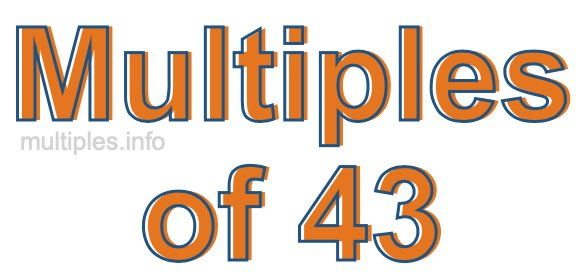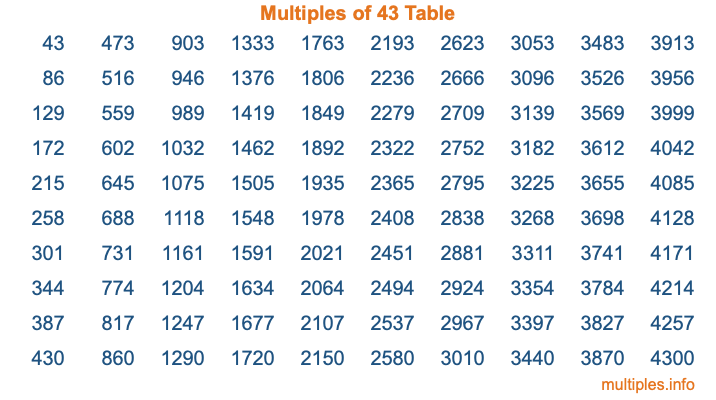Multiples of 43Welcome to the Multiples of 43 page. Here we will first teach you everything you will ever need to know about the multiples of 43, and then give you a study guide summary of everything we taught you to make sure you remember it all. Use this page to look up facts and learn information about the multiples of 43. This page will make you a multiples of forty-three expert!

Definition of Multiples of 43
Multiples of 43 are all the numbers that when divided by 43 equal an integer. Each of the multiples of 43 are called a multiple. A multiple of 43 is created by multiplying 43 by an integer.

Therefore, to create a list of multiples of 43, you start with 1 multiplied by 43, then 2 multiplied by 43, then 3 multiplied by 43, and so on for as long as you want. Thus, the list of the first five multiples of 43 is 43, 86, 129, 172, and 215. To see a larger list of multiples of 43, see the printable image of Multiples of 43 further down on this page. We also have a category where you can choose any nth multiple of 43.

Multiples of 43 Checker
The Multiples of 43 Checker below checks to see if any number of your choice is a multiple of 43. In other words, it checks to see if there is any number (integer) that when multiplied by 43 will equal your number. To do that, we divide your number by 43. If the the quotient is an integer, then your number is a multiple of 43.

Is  a multiple of 43?

Least Common Multiple of 43 and ...
A Least Common Multiple (LCM) is the lowest multiple that two or more numbers have in common. This is also called the smallest common multiple or lowest common multiple and is useful to know when you are adding our subtracting fractions. Enter one or more numbers below (43 is already entered) to find the LCM.

Check out our LCM Calculator if you need more details about the Least Common Multiple or if you need the LCM for different numbers for adding and subtraction fractions.

nth Multiple of 43
As we stated above, 43 is the first multiple of 43, 86 is the second multiple of 43, 129 is the third multiple of 43, and so on. Enter a number below to find the nth multiple of 43.

th multiple of 43

Multiples of 43 vs Factors of 43
43 is a multiple of 43 and a factor of 43, but that is where the similarities end. All postive multiples of 43 are 43 or greater than 43. All positive factors of 43 are 43 or less than 43.

Below is the beginning list of multiples of 43 and the factors of 43 so you can compare:

Multiples of 43: 43, 86, 129, 172, 215, etc.

Factors of 43: 1, 43

As you can see, the multiples of 43 are all the numbers that you can divide by 43 to get a whole number. The factors of 43, on the other hand, are all the whole numbers that you can multiply by another whole number to get 43.

It's also interesting to note that if a number (x) is a factor of 43, then 43 will also be a multiple of that number (x).

Multiples of 43 vs Divisors of 43
The divisors of 43 are all the integers that 43 can be divided by evenly. Below is a list of the divisors of 43.

Divisors of 43: 1, 43

The interesting thing to note here is that if you take any multiple of 43 and divide it by a divisor of 43, you will see that the quotient is an integer.

Multiples of 43 Table
Below is an image of the first 100 multiples of 43 in a table. The table is in chronological order, column by column. The first column has the first ten multiples of 43, the second column has the next ten multiples of 43, and so on.The Multiples of 43 Table is also referred to as the 43 Times Table or Times Table of 43. You are welcome to print out our table for your studies.

Negative Multiples of 43
Although not often discussed or needed in math, it is worth mentioning that you can make a list of negative multiples of 43 by multiplying 43 by -1, then by -2, then by -3, and so on, to get the following list of negative multiples of 43:

-43, -86, -129, -172, -215, etc.

Multiples of 43 Summary
Below is a summary of important Multiples of 43 facts that we have discussed on this page. To retain the knowledge on this page, we recommend that you read through the summary and explain to yourself or a study partner why they hold true.

There are an infinite number of multiples of 43.

A multiple of 43 divided by 43 will equal a whole number.

43 divided by a factor of 43 equals a divisor of 43.

The nth multiple of 43 is n times 43.

The largest factor of 43 is equal to the first positive multiple of 43.

43 is a multiple of every factor of 43.

43 is a multiple of 43.

A multiple of 43 divided by a divisor of 43 equals an integer.

43 divided by a divisor of 43 equals a factor of 43.

Any integer times 43 will equal a multiple of 43.

Multiples of a Number
Here you can get the multiples of another number, all with the same attention to detail as we did for multiples of 43 on this page.

Multiples of
Multiples of 44
Did you find our page about multiples of forty-three educational? Do you want more knowledge? Check out the multiples of the next number on our list!